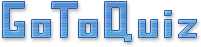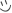# OpenStax Chemistry Chapter 2 Quiz

Here is a quiz to test your knowledge of the second chapter of Openstax's chemistry textbook. This quiz is intended for students of the high school level.

I am currently taking a chemistry class at a community college, so I am in no way perfect. If any information in this quiz is in any way incorrect, feel free to email me about it.

Created by: Finley Root

1. What were John Dalton's 4 postulates?
2. Thompson's cathode ray tube experiment determined:
3. The Millikan oil drop experiment determined:
4. Rutherford's gold foil experiment determined that
5. The charge of a proton, neutron, and electron are
6. The atomic number is
7. The mass number is
8. The charge of an atom can be calculated by
9. Ions are atoms with an imbalance of protons and (A). Cations are (B), and anions are negative.
10. Isotopes are variants of atoms that have differing numbers of
11. Atomic mass is found on the periodic table under an element's atomic symbol. What does this number represent?
12. How is 2NaHCo3 different from Na2HCo3?
13. Which of the following is methane's structural formula?
14. Sulfuric acid's molecular formula is H2SO4. What is sulfuric acid's empirical formula?
15. Isomers are
16. The mole is defined as
17. The molar mass is
18. Billy finds 189.97g of gold in his closet, how many moles of gold does Billy have? (Au atomic mass = 196.97 g/mol)
19. Susie dropped her 137.33 moles of Barium. How many grams of Barium did she drop? (Ba atomic mass = 137.33 g/mol)

You're about to get your result. Then try our new sharing options.